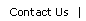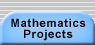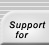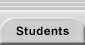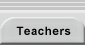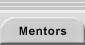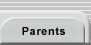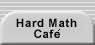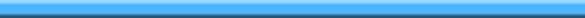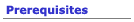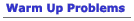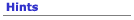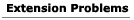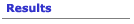Extensions for the Amida-kuji Problem

Efficiency: "Worst Case" Assignment

For an amida-kuji with a certain number of players, what is the maximum number of horizontal lines you would ever need to create an ordering?  If you think of horizontal lines as having a cost, that number is considered the "worst case" number.  What is the worst case for 2 players?; for 3 players?; for n players?

Sorting Networks

If each horizontal line is thought of as a "sorting element" that is activated only when the number on the left is greater than the number on the right, then a "network lottery" turns into a "sorting network."   Computer scientists have done quite a bit of research on sorting networks.  However, most networks normally allow comparisons between nonadjacent lines, as in the figure below.  It is the most efficient network that is know  for sorting 13 items.  The sorting networks we get from amida-kuji are planar sorting networks, because the networks can be drawn on the plane without any lines crossing.For non-planar sorting networks, see http://unplugged.canterbury.ac.nz/sortnet/index.htm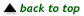Translations of mathematical formulas for web display were created by tex4ht. © Copyright 2003 Education Development Center, Inc. (EDC)